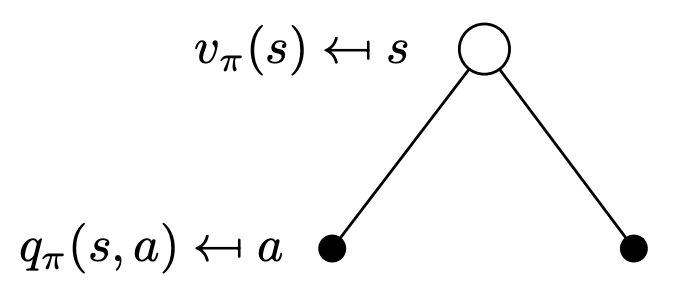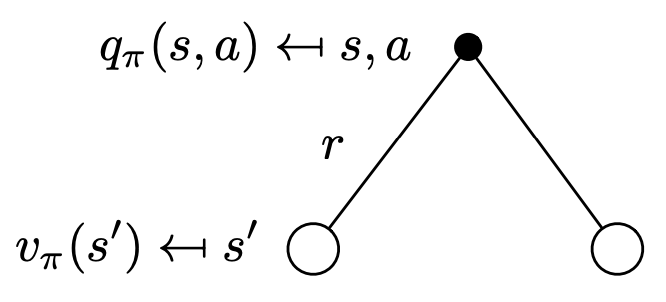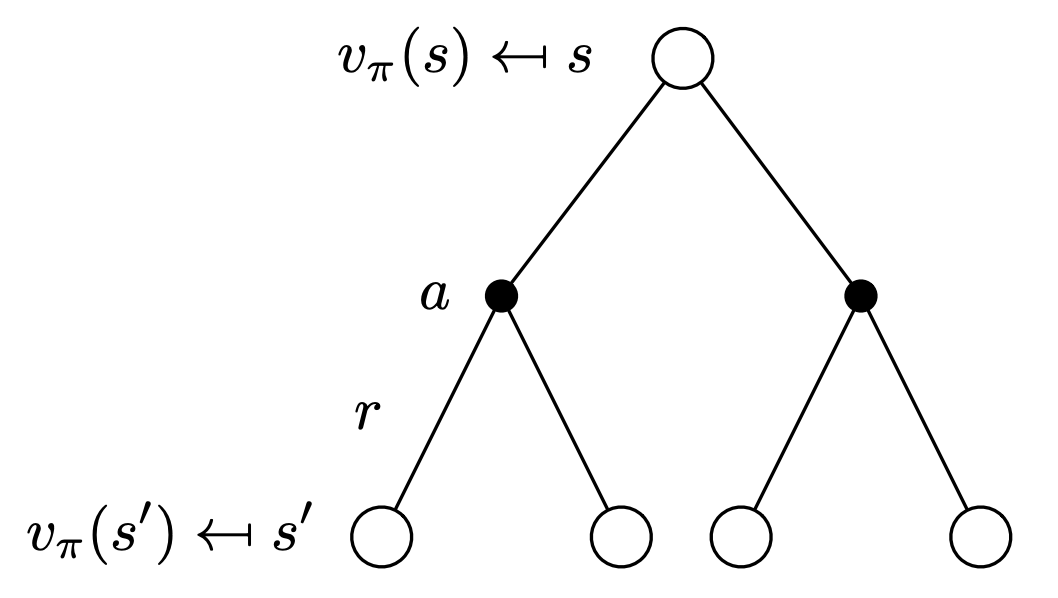# Value Function §

The Value function is used to estimate how good it is for the agent to be in a given state.

Used for Policy Evaluation.

Whereas the Reward indicates what is good in the immediate sense, the value function specifies what is good in the long run.

For an MRP, The value function gives the long-term value of state . They are defined in terms of a Policy.

Definition

The state-value function of an MDP is the expected Return starting from state , and then following policy

Definition

The action-value function of an MDP is the expected Return starting from state , taking action , and then following policy

But we can do better, because we are making assumptions that the problem we are solving is a MDP, so the current state has captured all information (not dependent on past rewards). We can break the equation into two parts (the idea of breaking our value function down into these 2 parts is called a Bellman Equation):

With the value function, the probability (weight of each edge) is given by the policy, we decide that. Below are called Backup Diagram:On the other hand, for the q-value (i.e. after we have done an action), this is what we do with the environment. After choosing an action, we get a reward, and the probability of landing into new states are given by our environment. We also apply the Discount Factor here.So now, we can have a recursive relationship.We use Bellman Equation to calculate the value function.

Important

The value function of the terminal state is always

### Computing the Value Function §

We can run a bunch of simulations and average the returns to compute the value function of a particular state, where you use the janky equation that you don’t usually use.

### State-Value vs. Action-Value Function §

Maybe I talked about this somewhere else, but in Model-Free Control, you ABSOLUTELY need to use action-value function , it will not work with , because that depends on the next value function? See Generalized Policy Iteration